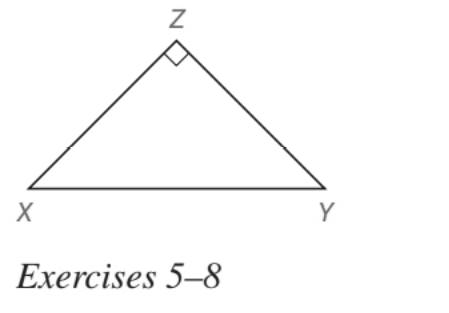Chapter 5.5, Problem 7E### Elementary Geometry for College St...

6th Edition
Daniel C. Alexander + 1 other
ISBN: 9781285195698

#### Solutions

Chapter
Section### Elementary Geometry for College St...

6th Edition
Daniel C. Alexander + 1 other
ISBN: 9781285195698
Textbook Problem
1 views

# In Exercises 5 to 22, find the missing lengths. Give your answers in both simplest radical form and as approximations correct two decimal places Given: Right ∆ X Y Z with X Z ¯ ≅ Y Z ¯ and X Y = 10 2 Find: X Z and Y ZTo determine

To find:

XZ and YZ for a right XYZ with XZ¯YZ¯ and XY=102.

Explanation

Approach:

For a right triangle for which the measure of the interior angles 45°, 45°, and 90°, if ‘a’ is the length of measure of one of the leg; opposite to the angle 45°, then the length of the other two sides is given by

Length of the other leg =a

Length of the hypotenuse =a2

In general

Length of the legs are equal.

Length of the hypotenuse =2× (Length of one of the legs)

Calculation:

Given,

A right XYZ with XZ¯YZ¯ and XY=102.

Since, two of the sides of the right triangle are congruent, the angles opposite to the sides must be equal and should be 45° each.

Thus, the XYZ become

Here, XY is the hypotenuse of the XYZ.

By the application of 45°-45°-90°

45°-45°-90° theorem

### Still sussing out bartleby?

Check out a sample textbook solution.

See a sample solution

#### The Solution to Your Study Problems

Bartleby provides explanations to thousands of textbook problems written by our experts, many with advanced degrees!

Get Started

#### Find more solutions based on key concepts